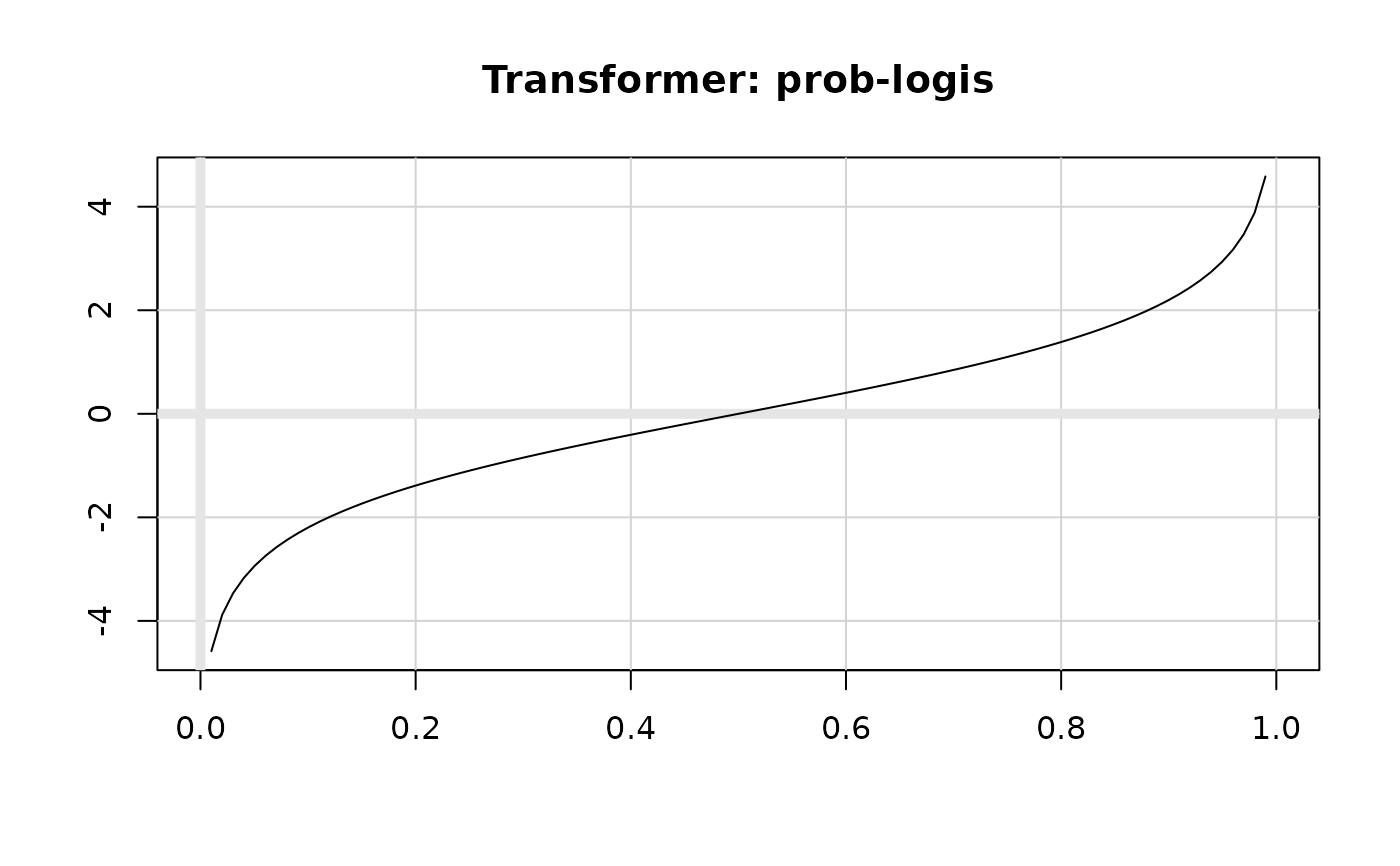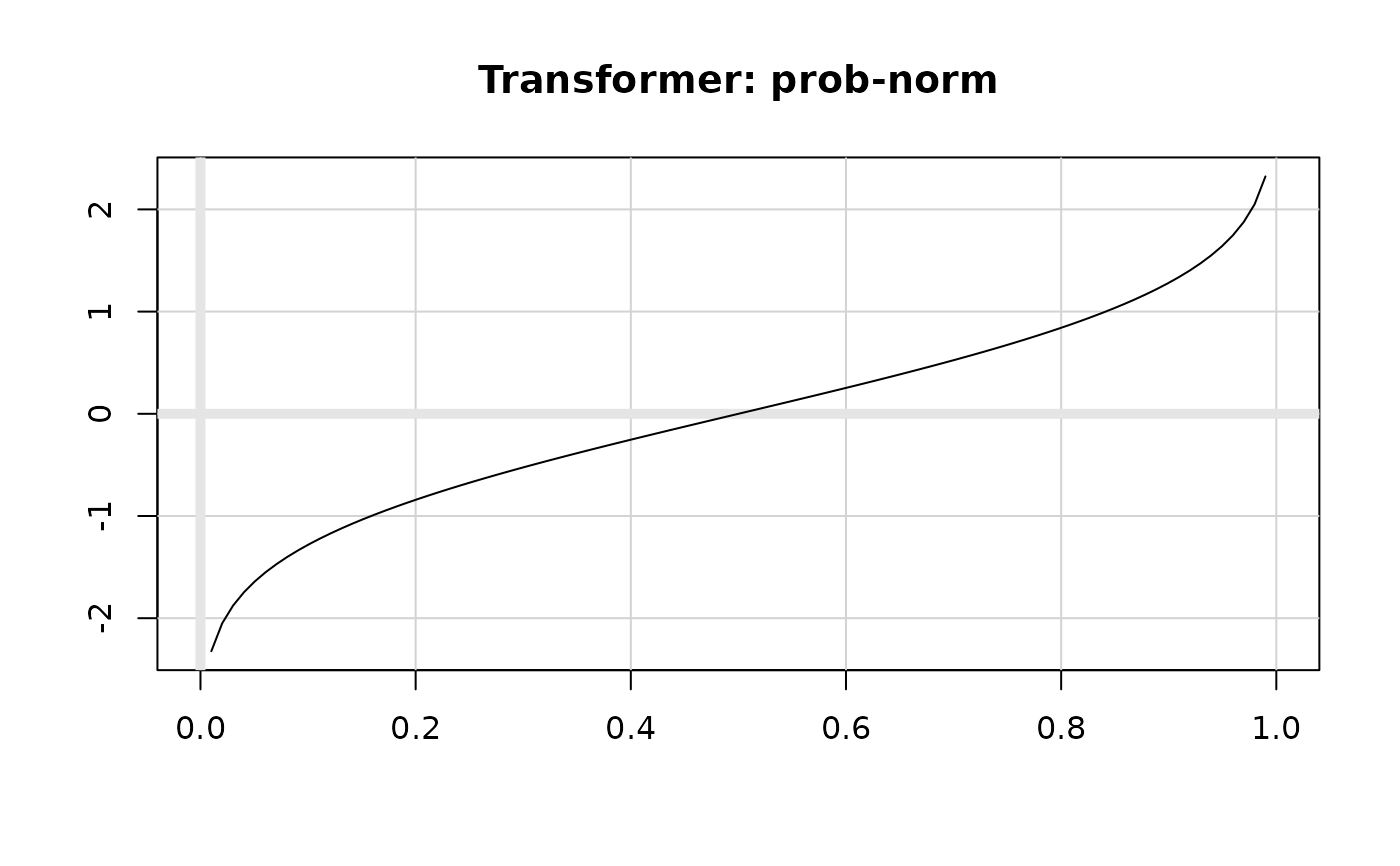Probability transformation

## Usage

probability_trans(distribution, ...)

logit_trans()

probit_trans()

## Arguments

distribution

probability distribution. Should be standard R abbreviation so that "p" + distribution is a valid probability density function, and "q" + distribution is a valid quantile function.

...

other arguments passed on to distribution and quantile functions

## Examples

plot(logit_trans(), xlim = c(0, 1))plot(probit_trans(), xlim = c(0, 1))